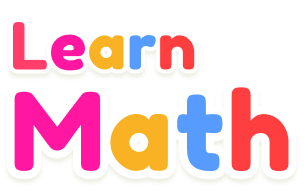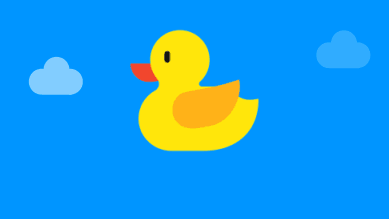•••Do you know the colors of these shapes?

•Can you count the ducks of different colors?

•Can you name these characters?

•Take you through addition within ten.

•Let us learn colors.

•Can you count the ducks of different colors?

•Do you recognize the geometric shapes on the train?

•Take you to know these villain numbers.

•Can you name these characters?

•••Can you count these animals?

•Learning colors and math with sharks

•Can you find the biggest duck?

•Could you find out the funny shapes?

•Come and learn numbers and colors with ice cream!

•Do you recognize the colors of these cars?

••Can you tell the different colors and numbers?

••Do you tell the numbers in colorful eggs?

•Do you know the names of these shapes?

•Do you know how to add and subtract within 10?

•Let's learn basic math subtraction!

•Can you distinguish the various colors of balloons?

•Let's do some simple calculations.

•Can you tell the right shapes of the following pictures?

•Do you know that magic changes shape?

•Let's get to know the shapes!

•Have you ever seen a geometric robot?

••Play a little game of choosing shapes.

•Which rope is longer? Get to know the tools of the test together.

•Do you know colors and numbers of these ice cream?

•can you figure out the numbers？

•Can you tell the right shapes of the following pictures?

•What is the color of the shapes?

•Do you know how many lollipops this is?

•Can you recognize the shapes of the different objects?

•Can you count the number of squares?

•Let's count some cars.

•Count how many slices of pizza there are?

•Let's count balloons

•Let's count the number of spots on the ladybug.

•Can you tell the different colors and numbers?

•Can you count the ducks of different colors?

•Can you tell the different colors and numbers?

•Let's count these excavator.

•Can you calculate mathematical addition and subtraction within 10?

•Let's learn basic math subtraction!

•What time is it?

••Can you tell different numbers?

•Could you find out the funny shapes?

•Let's get acquainted with geometric shapes

•Let's learn days of the week!

•Learn addition with the cute bunny!

•Fun Math Quiz!

•Can you distinguish the order of these fruits?

•Can you tell the right shapes of the following pictures?

•Do you know how many kittens there are?

•Can you count from 1 to 10?

•Do you know these algorithms?

•Let's count ducks together.

•Take you to know these villain numbers.

•Do you know if there is an odd or even number on the balloon?

•Let's learn basic math subtraction!

•Can you count from 1 to 10?

•Do you know these aquatic animals？

•Do you know these aquatic animals？

•Can you count the ducks of different colors?

•Can you distinguish the order of these animals?

•Follow the ducklings to learn colors and math.

•Can you count the ducks of different colors?

•Do you know these animals?

•Can you count the ducks of different colors?